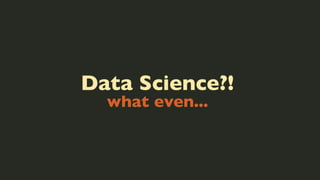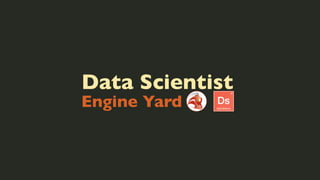Diese Präsentation wurde erfolgreich gemeldet.
Die SlideShare-Präsentation wird heruntergeladen. ×

# Data Science, what even?!Wird geladen in …3
×

1 von 120
1 von 120

# Data Science, what even?!

Presented an abridged version of my "What is data science" talk at #websummit 2013.

This talk goes over the required skillset as defined by Drew Conway and his famous venn diagram, and also outlines the Data Scientific Method brought by Dr. Patil. The talk is mainly two parts and the second part goes over some of the packages and technologies we use — minus the storage part.

Presented an abridged version of my "What is data science" talk at #websummit 2013.

This talk goes over the required skillset as defined by Drew Conway and his famous venn diagram, and also outlines the Data Scientific Method brought by Dr. Patil. The talk is mainly two parts and the second part goes over some of the packages and technologies we use — minus the storage part.

## Weitere Verwandte Inhalte

### Data Science, what even?!

1. 1. Data Science?! what even...
2. 2. David Coallier @davidcoallier
3. 3. Data Scientist Engine Yard
4. 4. And I cook.. A lot.
5. 5. (n-1) items
7. 7. Feedback.
8. 8. Indifference.
9. 9. Young mathematically inclined minds
10. 10. Young mathematically inclined minds We knew everything.
11. 11. First Bad Assumption.
12. 12. So we asked “experts”.
13. 13. Wrong Ingredients
14. 14. Bad Data
15. 15. Tasted like sh*t
16. 16. From Our Results We had questions.
17. 17. Found Expertise Not Online.
18. 18. Data Scientiﬁc Method
19. 19. Find a Question Your Hypothesis
20. 20. Current Data What do you have?
21. 21. Features & Tests Try it.
22. 22. Analyse Results Won’t be pretty.
23. 23. Conversation Framed. By. Data.
24. 24. But....
25. 25. Good Discussions Imply good data scientists
26. 26. Hacking Skills
27. 27. Hacking Skills Maths & Stats
28. 28. Hacking Skills Expertise Maths & Stats
29. 29. Hacking Skills Machine Learning Danger Zone!!! Expertise Research Maths & Stats
30. 30. Hacking Skills Data Science Expertise Maths & Stats
31. 31. Hacking Skills Danger Zone!!! Machine Learning Data Science Maths & Stats Expertise Research
32. 32. Business Don’t need an MBA
33. 33. In other words.
34. 34. 1. Hacking 2. Maths & Stats 3. Expertise
35. 35. Apply Method Data Scientiﬁc
36. 36. 1. Question 2. Current Data 3. Features/Tests 4. Analyse 5. Converse
37. 37. Find a Question Let’s imagine Github
38. 38. Upgrade Repos Affect users as little as possible
39. 39. import csv content = csv.read('repo1.csv')
40. 40. λ e f (k; λ ) = k! k −k for k >= 0
41. 41. Converse Present Findings
42. 42. Iterate Commits aren’t key.
43. 43. KPIs are key Indicators from experience
44. 44. Questions Super Important.
45. 45. Just test it..
46. 46. We are Human. Emotional Connection
47. 47. What next? Second Hypothesis.
48. 48. Focus on Data Relevant to your KPIs.
49. 49. Data gives you the what Humans give you the why
50. 50. Turn Information
51. 51. Into Actionable Insight
52. 52. Create Discussions Introspection Engines
53. 53. Seeing, Feeling it The brain sees.
54. 54. Not regressions
55. 55. Not p-values
56. 56. Not slopes
57. 57. Not F-statistics
58. 58. Not coefﬁcients
59. 59. Question Data Not Visualisations.
60. 60. Toolbox What do we use?
61. 61. R Modeling, Testing, Prototyping
62. 62. RStudio The IDE
63. 63. lubridate and zoo Dealing with Dates...
64. 64. yy/mm/dd mm/dd/yy YYYY-mm-dd HH:MM:ss TZ yy-mm-dd 1363784094.513425 yy/mm different timezone
65. 65. reshape2 Reshape your Data
66. 66. ggplot2 Visualise your Data
67. 67. RCurl, RJSONIO Find more Data
68. 68. HMisc Miscellaneous useful functions
69. 69. forecast Can you guess?
70. 70. garch Generalized Autoregressive Conditional Heteroskedasticity
71. 71. quantmod Statistical Financial Trading
72. 72. getSymbols('AAPL') barChart(AAPL) addMACD()
73. 73. xts Extensible Time Series
74. 74. igraph Study Networks
75. 75. maptools Read & View Maps
76. 76. map('state', region = c(row.names(USArrests)), col=cm.colors(16, 1)[ﬂoor(USArrests\$Rape/max(USArrests\$Rape)*28)], ﬁll=T)
77. 77. Python Scientiﬁc Computing
78. 78. SciPy http://www.scipy.org
79. 79. scipy.stats
80. 80. scipy.stats Descriptive Statistics
81. 81. from scipy.stats import describe s = [1,2,1,3,4,5] print describe(s)
82. 82. scipy.stats Probability Distributions
83. 83. Example Poisson Distribution
84. 84. λ e f (k; λ ) = k! k −k for k >= 0
85. 85. import scipy.stats.poisson p = poisson.pmf([1,2,3,4,1,2,3], 2)
86. 86. print p.mean() print p.sum() ...
87. 87. NumPy http://www.numpy.org/
88. 88. NumPy Linear Algebra
89. 89. ⎛ 1 0 ⎞ ⎜ 0 1 ⎟ ⎝ ⎠
90. 90. import numpy as np x = np.array([ [1, 0], [0, 1] ]) vec, val = np.linalg.eig(x) np.linalg.eigvals(x)
91. 91. >>> np.linalg.eig(x) ( array([ 1., 1.]), array([ [ 1., 0.], [ 0., 1.] ]) )
92. 92. Matplotlib Python Plotting
93. 93. statsmodels Advanced Statistics Modeling
94. 94. NLTK Natural Language Tool Kit
95. 95. scikit-learn Machine Learning
96. 96. from sklearn import tree X = [[0, 0], [1, 1]] Y = [0, 1] clf = tree.DecisionTreeClassifier() clf = clf.fit(X, Y) clf.predict([[2., 2.]]) >>> array()
97. 97. PyBrain ... Machine Learning
98. 98. PyMC Bayesian Inference
99. 99. Pattern Web Mining for Python
100. 100. NetworkX Study Networks
101. 101. MILK: Machine Learning
102. 102. Pandas easy-to-use data structures
103. 103. from pandas import * x = DataFrame([ {"age": 26}, {"age": 19}, {"age": 21}, {"age": 18} ]) print x[x['age'] > 20].count() print x[x['age'] > 20].mean()
104. 104. Python vs R? Different Purposes
105. 105. Dogfooding Data Scientiﬁc Method
106. 106. Original Question What is Data Science?
107. 107. Back to you For questioning